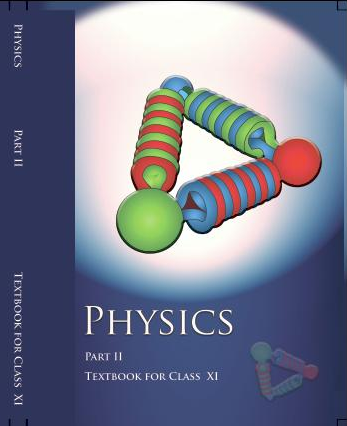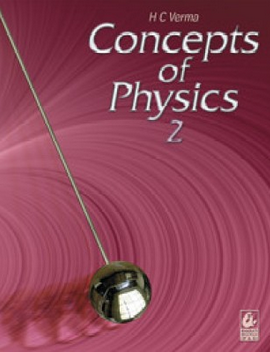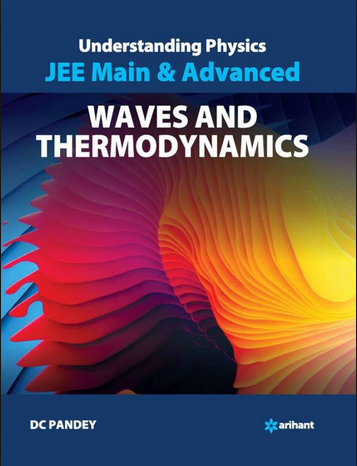# Thermodynamics   Share

## What is Thermodynamics

When you rub your hands for a few seconds, you will find that your palms get heated up and if you observe this phenomenon using spectacles of science, you will realize that basically mechanical energy (rubbing hands) is converted to heat energy (heated palms). Similarly, take the case of a steam engine, in which heat energy (steam) is converted into mechanical energy (movement). So basically in this chapter, you will read about different forms of energy and how one energy form is converted into another energy form.## Thermodynamics - Topics

Thermal equilibrium, zeroth law of thermodynamics, the concept of temperature, Heat, work and

internal energy, First law of thermodynamics, The second law of thermodynamics, reversible and, irreversible processes, Carnot engine, and its efficiency.

## Overview of Thermodynamics

In this chapter, you will come across 4 laws of thermodynamics, all the concepts and questions in this chapter revolve around these 4 laws. Let’s understand all of these laws briefly.

• Zeroth Law: This law tells about the concept of temperature, it says if two bodies/system are in thermal equilibrium (no heat transfer) with a third body then all of the systems are in thermal equilibrium with each other.

• First Law: The change in internal energy of a system is equal to the heat added to the system minus the work done by the system. This law also states that energy is always conserved, it can neither be created nor be destroyed, it can only be transferred from one energy form to another form.

• Second Law: In simple terms, it states that the entropy (disturbance) of a closed system always increases over time. For example, if we take the universe as a closed system, its entropy is always increasing.

• Third Law: It states that the entropy approaches a constant value when the temperature tends to absolute zero temp (0 K).

Too difficult to understand these laws? let's take help of some real-life examples to understand these laws, most of you must have observed that when you go out in very crowded places, you start sweating, this phenomenon involves both first law and the second law of thermodynamics, sweating occurs to cool down our body, what happens is that the sweat take heat from over body and then evaporates in the atmosphere and in that way our body cools down (1st law) but at the same time the evaporated sweat warms the surrounding leading to more warmness and in this way people continuously sweat. Hence you can observe that the warmness is increasing and it leads to an increase in entropy (2nd Law). Offcourse the system (crowded place) is not a closed system as the heat is also transferred to the environment, but for our understanding, we could take it as a nearly closed system.

Prepare Online for JEE Main/NEET

Crack JEE 2021 with JEE/NEET Online Preparation Program## Formulas for Thermodynamics

•• Efficiency of heat engine,• Efficiency of Carnot engine,• Coefficient of Performance,•;;## How to prepare Thermodynamics

In this chapter, you mainly have to first understand the different types of process like Isochoric, isobaric and adiabatic process and you should also give a lot of attention on the P-V, P-T, V-T diagrams of these processes, many questions are asked from these diagrams itself. Most of the questions will revolve around the concept of First law of thermodynamics so try to solve more questions from this important topic. Also, many questions will involve the ideal gas equation for solving questions, it is highly recommended to you to make a good understanding of the chapter Kinetic theory of gases. Without reading the Kinetic Theory of Gases, it would be very difficult for you to solve the questions.

## Tips for Thermodynamics

• Always keep in mind the sign conventions, the sign convention in physics are different than the chemistry for thermodynamics because in physics we take work done by the system as a positive entity while in Chemistry we take work done on the system as positive.

• Keep a list of state variables and process variables, and keep in mind the differences between these two variables example, Entropy, and Internal Energy are state variables while work is a process variable.

• Understand the different type of processes, like the isobaric, isothermal, adiabatic and isochoric process and learn to make diagrams of these process like P-V, P-T, and V-T etc.

• Keep revising notes and give regular mock tests for getting a good understanding of this chapter.

## Books for Thermodynamics

Recommendation for this chapter would be to first go through NCERT book and solve questions after that you should move on to NCERT Exemplar book for a good hold on this chapter. If you want to test yourself for competitive exams, then you should read Understanding Physics by D.C. Pandey or you could also read and practice questions from Concepts of Physics by H.C. Verma.

## Physics Chapter wise Notes For Engineering and Medical Exams

 Chapters No. Chapters Name Chapter 1 Physics and Measurement Chapter 2 Kinematics Chapter 3 Laws of motion Chapter 4 Work Energy and Power Chapter 5 Rotational Motion Chapter 6 Gravitation Chapter 7 Properties of Solids and Liquids Chapter 8 Kinetic theory of Gases Chapter 10 Oscillations and Waves Chapter 11 Electrostatics Chapter 12 Current Electricity Chapter 13 Magnetic Effects of Current and Magnetism Chapter 14 Electromagnetic Induction and Alternating currents Chapter 15 Electromagnetic Waves Chapter 16 Optics Chapter 17 Dual Nature of Matter and Radiation Chapter  18 Atoms And Nuclei Chapter 19 Electronic devices Chapter 20 Communication Systems Chapter 21 Experimental skills

### Topics from Thermodynamics

• Thermal equilibrium, zeroth law of thermodynamics, the concept of temperature ( JEE Main ) (13 concepts)
• First law of thermodynamics. ( JEE Main ) (5 concepts)
• Heat ( JEE Main ) (4 concepts)
• work ( JEE Main ) (4 concepts)
• internal energy ( JEE Main ) (5 concepts)
• reversible and irreversible processes ( JEE Main ) (35 concepts)
• Carnot engine and its efficiency. ( JEE Main ) (4 concepts)
• The second law of thermodynamics ( JEE Main ) (9 concepts)
• Second Law of Thermodynamics ( JEE Main ) (5 concepts)
• Thermodynamics Processes ( JEE Main ) (7 concepts)
• Introduction to Thermodynamics ( JEE Main ) (3 concepts)
• First law of thermodynamics ( JEE Main ) (2 concepts)

### Important Books for Thermodynamics

•••Exams
Articles
Questions